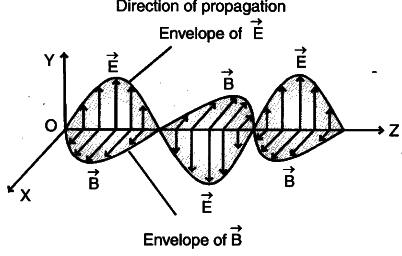# How does a charge q oscillating at certain frequency produce electromagnetic waves

How does a charge q oscillating at certain frequency produce electromagnetic waves ? Sketch a schematic diagram, depicting electric and magnetic fields for an electromagnetic wave propagating along the Z-direction.

1 Like

An electric charge at rest has an electric field in the region around it, but no magnetic field. A moving charge, however, produces both electric and magnetic fields. If the charge is moving with constant velocity (that is, the current is not changing with time), the fields will not change with time and no electromagnetic wave can be produced. If, however, the motion of the charge is accelerated, the electric and the magnetic fields will change with space and time; then it produces electromagnetic waves. Hence, we conclude that an accelerated charge emits electromagnetic waves.
In an oscillatory L-C circuit, a charge oscillates across the capacitor plates. This oscillating charge has a non-zero acceleration; hence it emits electromagnetic waves of frequency same as that of the oscillating charge.
The figure shows the graphical representation of an electromagnetic wave in which the electric field vector \overrightarrow{E} and the magnetic field vector \overrightarrow{B} are
vibrating along Y and X-directions respectively, and the wave is propagating along Z-direction. Both \overrightarrow{E} and \overrightarrow{B} vary with time and space and have the same frequency.1 Like

thank you so much